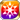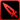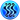# Not Quite Sweet

Type
Equipment
Rank
5★
Max level
40
Enhancer cost
2000
Enhancer EXP
20000
Vision Clear Reward
Source
Time Limited Summon
Release dates
• JP: 2019-02-14
• GL: 2020-02-13
AlchemistCodeDBRocking her yellow lily hair clip side to side, Itsuki really seems to enjoy the extra spicy chocolate. It was way too spicy for me. "To each their own, I guess." Yeah, there are all kinds of people in this world, and I think it would be fun to find all the different ways to enjoy chocolate, so that everyone could be as happy as Itsuki is right now. I wish I could watch her smile for just a little longer, but my eyes are starting to sting from all the spice in the air. You're so impressive, Itsuki... (Eve's chocolate recipe notes)

## Stats

#### Simple stats

TypeLvl 1Lvl 30Lvl 40
PATK+10+24+30

LevelPATK
1+10
2+10
3+11
4+11
5+12
6+12
7+13
8+13
9+14
10+14
LevelPATK
11+15
12+15
13+16
14+16
15+17
16+17
17+18
18+18
19+19
20+19
LevelPATK
21+20
22+20
23+21
24+21
25+22
26+22
27+23
28+23
29+24
30+24
LevelPATK
31+25
32+25
33+26
34+26
35+27
36+27
37+28
38+28
39+29
40+30

## Group skills

#### Simple stats

TypeLvl 1Lvl 30Lvl 40
Strong vs Fire+8+19+24

#### Detailed stats

LevelStrong vs Fire
1+8
2+8
3+8
4+9
5+9
6+10
7+10
8+10
9+11
10+11
LevelStrong vs Fire
11+12
12+12
13+12
14+13
15+13
16+14
17+14
18+14
19+15
20+15
LevelStrong vs Fire
21+16
22+16
23+17
24+17
25+17
26+18
27+18
28+19
29+19
30+19
LevelStrong vs Fire
31+20
32+20
33+21
34+21
35+21
36+22
37+22
38+23
39+23
40+24

#### Limit Break

TypeLB1LB2LB3LB4LB5
Strong vs Fire+3+5+8+11+14

#### Simple stats

TypeLvl 1Lvl 30Lvl 40
Strong vs Fire+8+19+24

#### Detailed stats

LevelStrong vs Fire
1+8
2+8
3+8
4+9
5+9
6+10
7+10
8+10
9+11
10+11
LevelStrong vs Fire
11+12
12+12
13+12
14+13
15+13
16+14
17+14
18+14
19+15
20+15
LevelStrong vs Fire
21+16
22+16
23+17
24+17
25+17
26+18
27+18
28+19
29+19
30+19
LevelStrong vs Fire
31+20
32+20
33+21
34+21
35+21
36+22
37+22
38+23
39+23
40+24

#### Limit Break

TypeLB1LB2LB3LB4LB5
Strong vs Fire+3+5+8+11+14

### Sweet and Spicy

#### Simple stats

TypeLvl 1Lvl 30Lvl 40
AGI+3+6+8
DEX+20+49+60
PDEF+10+24+30

LevelAGIDEXPDEF
1+3+20+10
2+3+21+10
3+3+22+11
4+3+23+11
5+3+24+12
6+3+25+12
7+3+26+13
8+3+27+13
9+4+28+14
10+4+29+14
LevelAGIDEXPDEF
11+4+30+15
12+4+31+15
13+4+32+16
14+4+33+16
15+4+34+17
16+4+35+17
17+5+36+18
18+5+37+18
19+5+38+19
20+5+39+19
LevelAGIDEXPDEF
21+5+40+20
22+5+41+20
23+5+42+21
24+5+43+21
25+6+44+22
26+6+45+22
27+6+46+23
28+6+47+23
29+6+48+24
30+6+49+24
LevelAGIDEXPDEF
31+6+50+25
32+6+51+25
33+7+52+26
34+7+53+26
35+7+54+27
36+7+55+27
37+7+56+28
38+7+57+28
39+7+58+29
40+8+60+30

#### Limit Break

TypeLB1LB2LB3LB4LB5
AGI+1+2+3+4+5
DEX+9+15+22+29+36
PDEF+5+8+11+14+18

### Yellow Lily Wish

#### Simple stats

TypeLvl 1Lvl 30Lvl 40
HP+120+328+400
Single Target ATK+4+9+12

#### Detailed stats

LevelHPSingle Target ATK
1+120+4
2+127+4
3+134+4
4+141+4
5+148+4
6+155+5
7+163+5
8+170+5
9+177+5
10+184+5
LevelHPSingle Target ATK
11+191+6
12+198+6
13+206+6
14+213+6
15+220+6
16+227+7
17+234+7
18+242+7
19+249+7
20+256+7
LevelHPSingle Target ATK
21+263+8
22+270+8
23+277+8
24+285+8
25+292+8
26+299+9
27+306+9
28+313+9
29+321+9
30+328+9
LevelHPSingle Target ATK
31+335+10
32+342+10
33+349+10
34+356+10
35+364+10
36+371+11
37+378+11
38+385+11
39+392+11
40+400+12

#### Limit Break

TypeLB1LB2LB3LB4LB5
HP+48+96+144+192+240
Single Target ATK+1+2+4+5+7

#### Max Limit Break

TypeLB5
Jewels Obtained+7

## Vision abilities

### Frost's Bite

#### Ability base

• Slot:Action
• DMG Type:Physical
• ATK Type:Slash
• Element:Water

After moving, Water Slash ATK on enemy unit & greatly raises own AGI until next turn [Range: 5, Height Range: 2]

Skill Effect
TypeMinMax
Attack80%120%
• Charges: 3
• Jewel Cost: 22
• Height: 2
• Select Range: Diamond
• Range: 0-5

#### Ability max

• Slot:Action
• DMG Type:Physical
• ATK Type:Slash
• Element:Water

After moving, Water Slash ATK on enemy unit & greatly raises own AGI until next turn [Range: 6, Height Range: 2]

Skill Effect
TypeMinMax
Attack160%160%
• Charges: 3
• Jewel Cost: 15
• Height: 2
• Select Range: Diamond
• Range: 0-6

### Limit Reached Bonus

#### Ability base

• Slot:Support

Revealed after Limit Reached. Raises AGI after appearing on map.

#### Ability max

• Slot:Action

Raises AGI after appearing on map.